# 13.2XFastTrie: Searching in Doubly-Logarithmic Time

The performance of the BinaryTrie structure is not that impressive. The number of elements,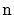, stored in the structure is at most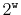, so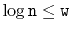. In other words, any of the comparison-based SSet structures described in other parts of this book are at least as efficient as a BinaryTrie, and don't have the restriction of only being able to store integers.

Next we describe the XFastTrie, which is just a BinaryTrie withhash tables--one for each level of the trie. These hash tables are used to speed up theoperation totime. Recall that theoperation in a BinaryTrie is almost complete once we reach a node,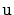, where the search path for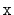would like to proceed to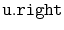(or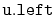) buthas no right (respectively, left) child. At this point, the search uses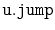to jump to a leaf,, of the BinaryTrie and either returnor its successor in the linked list of leaves. An XFastTrie speeds up the search process by using binary search on the levels of the trie to locate the node.

To use binary search, we need a way to determine if the nodewe are looking for is above a particular level,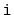, of ifis at or below level. This information is given by the highest-orderbits in the binary representation of; these bits determine the search path thattakes from the root to level. For an example, refer to Figure 13.6; in this figure the last node,, on search path for 14 (whose binary representation is 1110) is the node labelled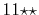at level 2 because there is no node labelled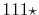at level 3. Thus, we can label each node at levelwith an-bit integer. Then the nodewe are searching for is at or below levelif and only if there is a node at levelwhose label matches the highest-orderbits of.In an XFastTrie, we store, for each, all the nodes at levelin a USet,, that is implemented as a hash table (). Using this USet allows us to check in constant expected time if there is a node at levelwhose label matches the highest-orderbits of. In fact, we can even find this node using.

The hash tables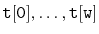allow us to use binary search to find. Initially, we know thatis at some levelwith. We therefore initialize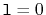and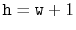and repeatedly look at the hash table, where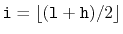. Ifcontains a node whose label matches's highest-orderbits then we set(is at or below level), otherwise we set(is above level). This process terminates when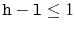, in which case we determine thatis at level. We then complete theoperation usingand the doubly-linked list of leaves.

    T find(T x) {
int l = 0, h = w+1, ix = it.intValue(x);
Node v, u = r, q = newNode();
while (h-l > 1) {
int i = (l+h)/2;
q.prefix = ix >>> w-i;
if ((v = t[i].find(q)) == null) {
h = i;
} else {
u = v;
l = i;
}
}
if (l == w) return u.x;
Node pred = (((ix >>> w-l-1) & 1) == 1) ? u.jump : u.jump.child;
return (pred.child[next] == dummy) ? null : pred.child[next].x;
}

Each iteration of theloop in the above method decreasesby roughly a factor of 2, so this loop findsafteriterations. Each iteration performs a constant amount of work and oneoperation in a USet, which takes constant expected time. The remaining work takes only constant time, so themethod in an XFastTrie takes onlyexpected time.

Theandmethods for an XFastTrie are almost identical to the same methods in a BinaryTrie. The only modifications are for managing the hash tables,...,. During theoperation, when a new node is created at level, this node is added to. During aoperation, when a node is removed form level, this node is removed from. Since adding and removing from a hash table take constant expected time, this does not increase the running times ofandby more than a constant factor. We omit a code listing orandsince it is almost identical to the (long) code listing already provided for the same methods in a BinaryTrie.

The following theorem summarizes the performance of an XFastTrie:

Theorem 13..2   An XFastTrie implements the SSet interface for-bit integers. An XFastTrie supports the operations
•andintime per operation and
•intime per operation.
The space used by an XFastTrie that storesvalues is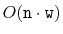.

opendatastructures.org